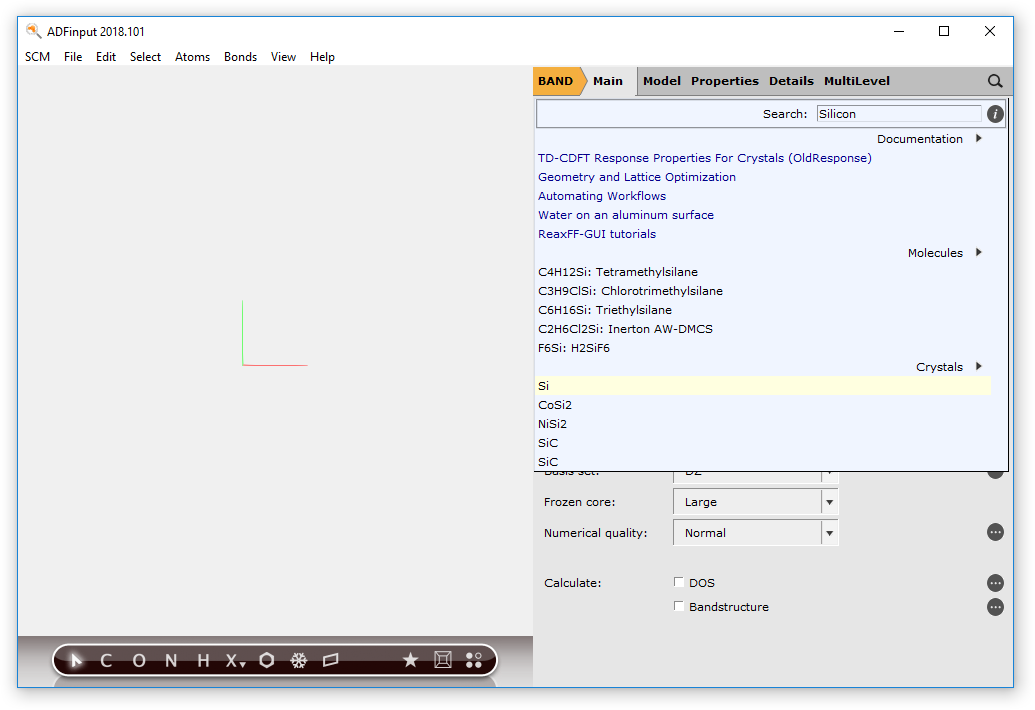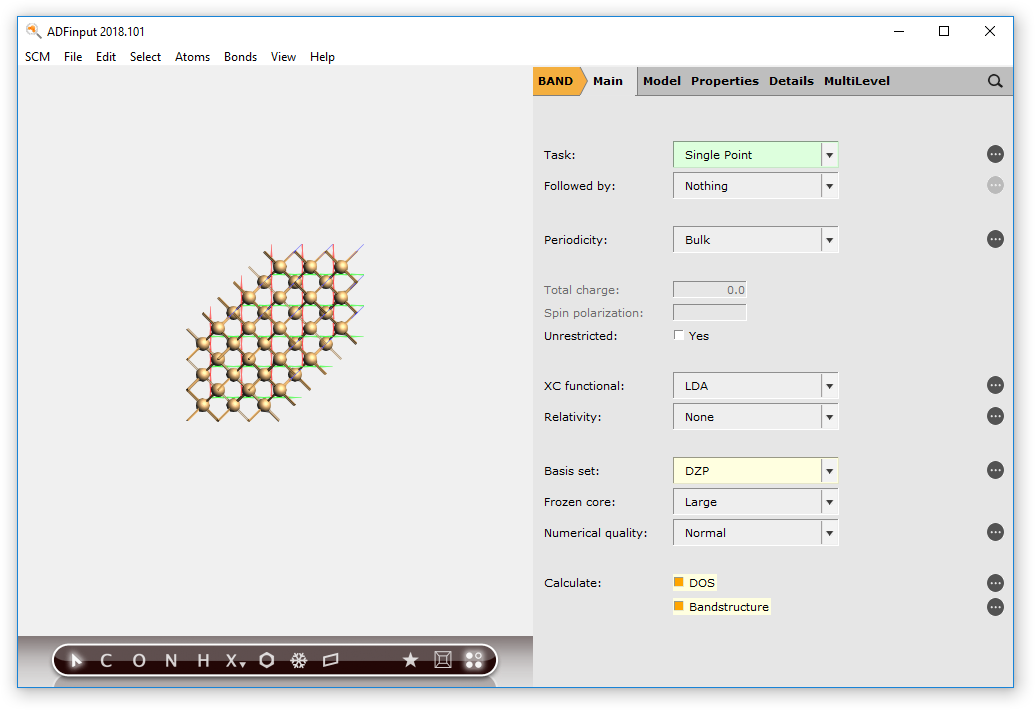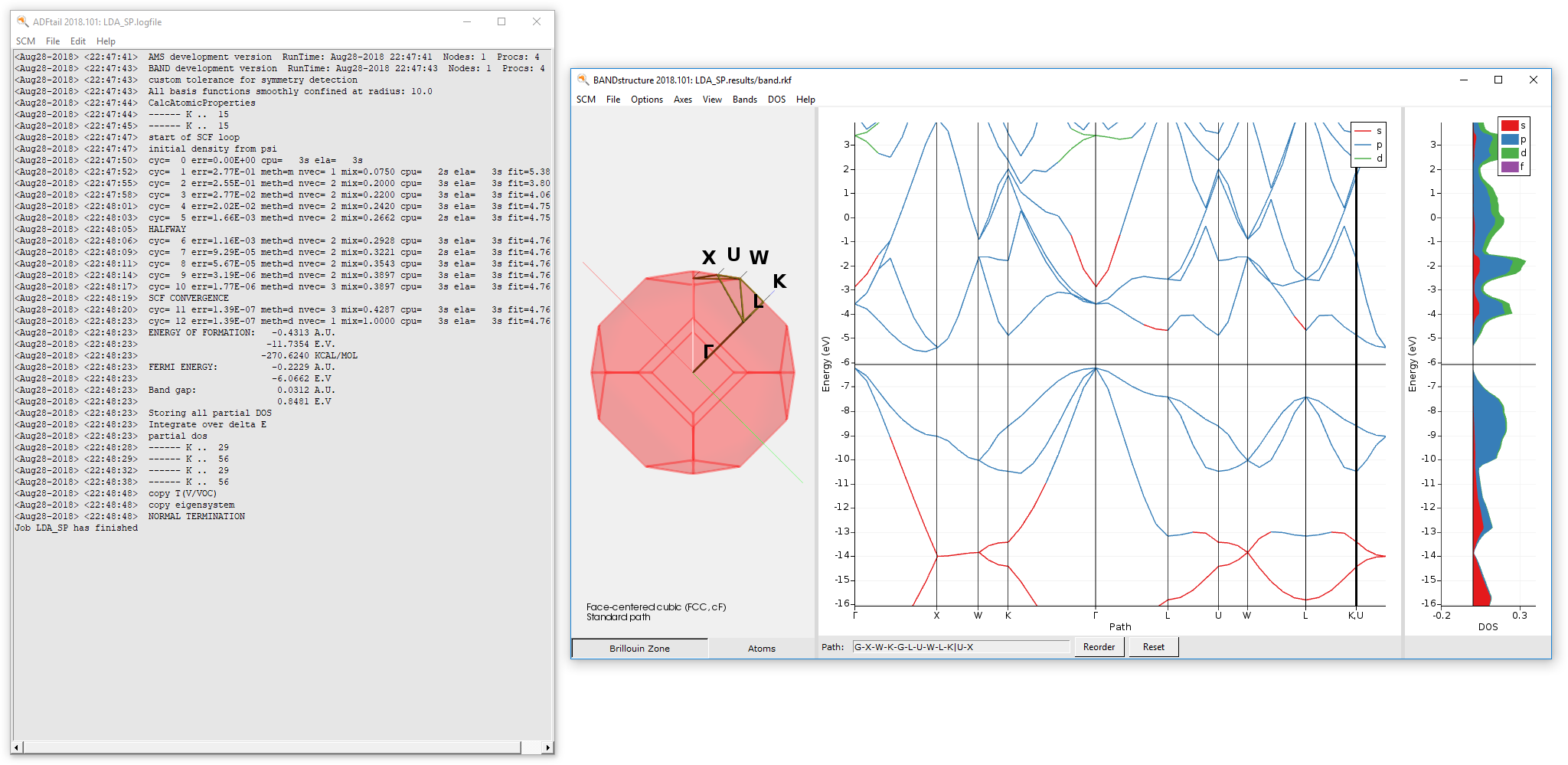# TD-CDFT Response Properties For Crystals (OldResponse)¶

BAND can calculate response properties such as the frequency-dependent dielectric function within the theoretical framework of time-dependent current density function theory (TD-CDFT).

This introductory tutorial will show you how to:

• Set up and run a BAND single point calculation (using ADFJobs and ADFInput)
• Set up and run a BAND TD-CDFT, linear response calculation (using ADFJobs and ADFInput) with the OldResponse method
• Visualize the dielectric function using ADFSpectra

If you are not at all familiar with our Graphical User Interface (GUI), check out the Introductory tutorial first.

## Step 1: Create the system¶

We now want to create a silicon crystal. Let us import the geometry from our database of structures:

2. Switch from ADF to BAND.
3. Search for Silicon in the search box (magnifying glass).
4. Select Crystals → Si.
5. click on View → Periodic → Repeat Unit Cells## Step 2: Run a Single Point Calculation (LDA)¶

We will first perform a single-point calculation of our Silicon crystal using LDA.

Tip

It is good practice to do a convergence study w. r. t. k-space sampling and basis set.

1. Select the ‘BAND Main’ panel.
2. Change Basis set to DZP.
3. Check the Bandstructure and DOS boxes.4. Go to Details → K-Space Integration
5. Set the K-Space grid type option to Symmetric
6. Set the Accuracy to 3.7. File → Save As..., use name LDA_SP.adf
8. File → Run

After the calculation finished, we can check the band-gap energy e.g. in the logfile. Furthermore, with the help of the BandStructure module we can validate that a very basic property is reproduced by this rather poor k-space sampling - Si diamond has an indirect band-gap.

9. SCM → Logfile
10. SCM → Bandstructure## Step 3: Run an OldResponse Calculation (ALDA)¶

We will now calculate the frequency-dependent dielectric function using linear response TD-CDFT.

In the previous step we learned that the calculated band-gap for the chosen theoretical level is approx. 0.85 eV. This is approximately 0.3 eV below the experimental band-gap. Hence, we will shift the virtual crystal orbitals by this value in energy space. We will sample the frequency range from 2.0 eV to 5.0 eV with a step-size of 0.1 eV.

1. Go to the ‘BAND Main’ panel and un-tick the Bandstructure and DOS check-boxes.
2. Go to Properties → Dielectric Function (TD-CDFT).
3. Change Method to OldResponse.
4. Change Number of frequencies to 31.
5. Change Starting frequency to 2.0.
6. Change End frequency to 5.0.
7. Change Shift to 0.3.8. File → Save As..., use name LDA_TDCDFT.adf
9. File → Run

After the calculation finished, we can visualize the dielectric function using ADFspectra.

10. SCM → Spectra
11. File → TD-CDFT → Dielectric Function → XXThe general features of the frequency-dependent dielectric function are nicely reproduced, but for a quantitatively better result one has to converge the k-space sampling, basis set and numerical integration. Also switching to the Berger2015 kernel would improve the results further. [Ref]